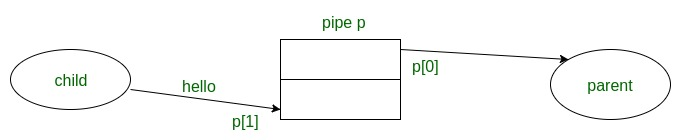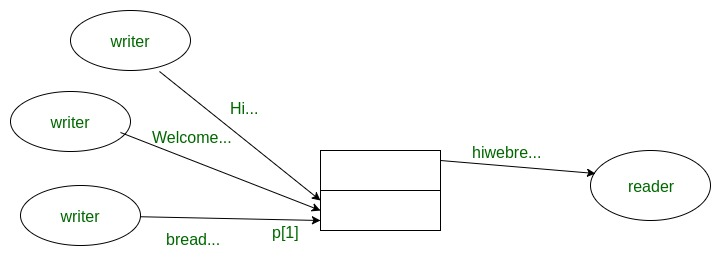# Non-blocking I/O with pipes in C

Prequisite: pipe() System call

When I/O block in pipe() happens?
Consider two processes, one process that’s gathering data(read data) in real time and another process that’s plotting it(write data). The two processes are connected by a pipe, with the data acquisition process feeding the data plotting process. Speed of the data acquisition of two process is different.
The default behavior in a pipe is for the writing and reading ends of a pipe is to exhibit blocking behavior, if the partner process is slower. This is bad because the data acquisition process can wait on the plotting process(write data). So, during the data acquisition process, block read call in pipe and program hangs. If we want this not to happen, we must close the write end in process before read end call.
In simple language,

• A read call gets as much data as it requests or as much data as the pipe has, whichever is less
• If the pipe is empty
• Reads on the pipe will return EOF (return value 0) if no process has the write end open
• If some process has the pipe open for writing, read will block in anticipation of new data

Non-blocking I/O with pipes

Sometimes it’s convenient to have I/O that doesn’t block i.e we don’t want a read call to block on one in case of input from the other. Solution for this is the given function:

```  To specify non-blocking option:
#include<fcntl.h>
int fd;
fcntl(fd, F_SETFL, O_NONBLOCK);
```
Parameter:

• fd: file descriptor
• F_SETFL: Set the file status flags to the value specified by arg. File access mode here in our purpose use only for O_NONBLOCK flag.
• O_NONBLOCK: use for non-blocking option.
Returns:

• 0: return on successful
• -1: return on error, set errorno

After this function runs successfully, a call to read/write returns -1 if pipe
is empty/full
and sets errno to EAGAIN

Example: Child writes “hello” to parent every 3 seconds and Parent does a non-blocking read each second.`// C program to illustrate ` `// non I/O blocking calls ` `#include ` `#include ` `#include // library for fcntl function ` `#define MSGSIZE 6 ` `char``* msg1 =“hello”; ` `char``* msg2 =“bye !!”; ` ` `  `int` `main() ` `{ ` `    ``int` `p, i; ` ` `  `    ``// error checking for pipe ` `    ``if` `(pipe(p) < 0) ` `        ``exit``(1); ` ` `  `    ``// error checking for fcntl ` `    ``if` `(fcntl(p, F_SETFL, O_NONBLOCK) < 0) ` `        ``exit``(2); ` ` `  `    ``// continued ` `    ``switch` `(fork()) { ` ` `  `    ``// error ` `    ``case` `-1: ` `        ``exit``(3); ` ` `  `    ``// 0 for child process ` `    ``case` `0: ` `        ``child_write(p); ` `        ``break``; ` ` `  `    ``default``: ` `        ``parent_read(p); ` `        ``break``; ` `    ``} ` `    ``return` `0; ` `} ` `void` `parent_read(``int` `p[]) ` `{ ` `    ``int` `nread; ` `    ``char` `buf[MSGSIZE]; ` ` `  `    ``// write link ` `    ``close(p); ` ` `  `    ``while` `(1) { ` ` `  `        ``// read call if return -1 then pipe is ` `        ``// empty because of fcntl ` `        ``nread = read(p, buf, MSGSIZE); ` `        ``switch` `(nread) { ` `        ``case` `-1: ` ` `  `            ``// case -1 means pipe is empty and errono ` `            ``// set EAGAIN ` `            ``if` `(``errno` `== EAGAIN) { ` `                ``printf``(“(pipe empty)\n”); ` `                ``sleep(1); ` `                ``break``; ` `            ``} ` ` `  `            ``else` `{ ` `                ``perror``(“read”); ` `                ``exit``(4); ` `            ``} ` ` `  `        ``// case 0 means all bytes are read and EOF(end of conv.) ` `        ``case` `0: ` `            ``printf``(“End of conversation\n”); ` ` `  `            ``// read link ` `            ``close(p); ` ` `  `            ``exit``(0); ` `        ``default``: ` ` `  `            ``// text read ` `            ``// by default return no. of bytes ` `            ``// which read call read at that time ` `            ``printf``(“MSG = % s\n”, buf); ` `        ``} ` `    ``} ` `} ` `void` `child_write(``int` `p[]) ` `{ ` `    ``int` `i; ` ` `  `    ``// read link ` `    ``close(p); ` ` `  `    ``// write 3 times "hello" in 3 second interval ` `    ``for` `(i = 0; i < 3; i++) { ` `        ``write(p, msg1, MSGSIZE); ` `        ``sleep(3); ` `    ``} ` ` `  `    ``// write "bye" one times ` `    ``write(p, msg2, MSGSIZE); ` ` `  `    ``// here after write all bytes then write end ` `    ``// doesn't close so read end block but ` `    ``// because of fcntl block doesn't happen.. ` `    ``exit``(0); ` `} `

Output:

```(pipe empty)
MSG=hello
(pipe empty)
(pipe empty)
(pipe empty)
MSG=hello
(pipe empty)
(pipe empty)
(pipe empty)
MSG=hello
(pipe empty)
(pipe empty)
(pipe empty)
MSG=bye!!
End of conversation
```

Atomic writes in pipe

Atomic means no other process ever observes that its partially done. Reading or writing pipe data is atomic if the size of data written is not greater than PIPE_BUF(4096 bytes). This means that the data transfer seems to be an instantaneous unit means nothing else in the system can observe a state in which it is partially complete. Atomic I/O may not begin right away (it may need to wait for buffer space or for data), but once it does begin it finishes immediately.

Data Writes of up to PIPE_BUF (4096 Bytes) are atomic. Reading or writing a larger amount of data may not be atomic; For example, output data from other processes sharing the descriptor may be interspersed. Also, once PIPE_BUF characters have been written, further writes will block until some characters are read

Example: Process 1 sends a 100-byte message at the same time, process 2 sends a 100-byte message No guarantee about the order, but pipe will receive all of one message followed by all of the other.

In non atomic writes for larger writes there is no such guarantee, data could get confusingly intermingled like, this:Pipe Capacity

• A pipe can hold a limited number of bytes.
• Writes fill the pipe and block when the pipe is full
• They block until another process reads enough data at the other end of the pipe and return when all the data given to write have been transmitted
• Capacity of a pipe is at least 512 bytes, usually more (system dependent)

 `// C program to illustrate ` `// finding capacity of pipe ` `#include ` `#include ` `#include ` `int` `count = 0; ` ` `  `// SIGALRM signal handler ` `void` `alrm_action(``int` `signo) ` `{ ` `    ``printf``(``"Write blocked after %d characters\n"``, count); ` `    ``exit``(0); ` `} ` `int` `main() ` `{ ` `    ``int` `p; ` `    ``char` `c = ``'x'``; ` ` `  `    ``// SIGALRM signal ` `    ``signal``(SIGALRM, alrm_action); ` ` `  `    ``// pipe error check ` `    ``if` `(pipe(p) == -1) ` `        ``exit``(1); ` ` `  `    ``while` `(1) { ` `        ``alarm(5); ` ` `  `        ``// write 'x' at one time when capacity full ` `        ``// write() block and after 5 second alarm ` `        ``write(p, &c, 1); ` ` `  `        ``// send signal and alrm_action handler execute. ` `        ``++count; ` `        ``alarm(0); ` `    ``} ` `} `

Output:

```Write blocked after 65536 characters
//output depend on the system so output may change in different system
```

Here, in while loop first 5 second alarm is set after write() call writes one character ‘x’ in the pipe. And count variable is used for count character write in the pipe. strong>alarm(0) means cancel the set alarm of 5 second. After some time when pipe capacity is full then write() call is block and program does not execute next instruction, after 5 second set alarm ringing and send signal SIGALRM. After that alram_action handler executes and prints how many maximum character can be written by pipe.